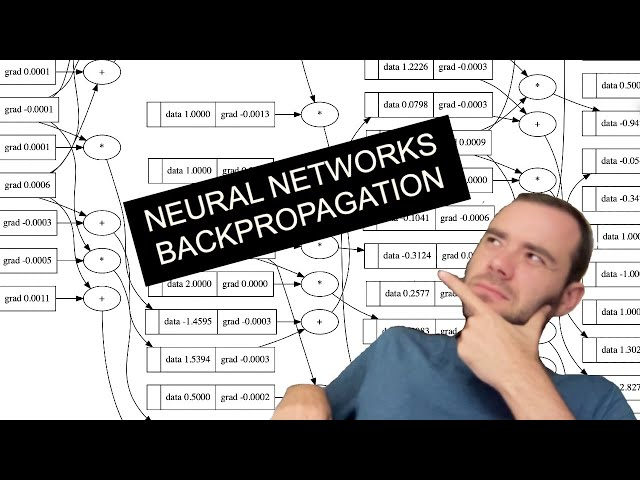# Learn Neural Networks & Backpropagation with Python & PyTorchTLDR Using Python and PyTorch, we can create and train a neural network with a forward pass, backward pass, and gradient descent update.

### Backpropagation and its importance in neural network training

• 🤯
Backpropagation allows us to evaluate the derivative of the output with respect to the inputs, giving us important information about how the inputs affect the output through the mathematical expression.
• 🧮
Back propagation involves calculating the gradient along all intermediate values, including the derivative of the loss function with respect to the weights of a neural network.
• 🤖
Backpropagation allows for optimization of neural networks by recursively applying the chain rule backwards through the computation graph.
• 🤯
The backward function can be customized to propagate gradients and chain rule at each node, making it possible to compute complex neural networks.
• 🧠
The trick used in deep learning to improve neural net performance is to calculate a single number that measures the total performance, called the loss.
• 🧠
Backpropagation allows us to calculate the gradient for every single parameter in the neural network, giving us the ability to nudge them a tiny amount based on the gradient information to minimize loss.

### Micrograd and its role in building and optimizing neural networks

• 🧠
Building micrograd allows for an intuitive understanding of how neural network training works under the hood, which is crucial for developing more advanced AI technologies.
• 💻
Micrograd is what you need to train your networks and everything else is just efficiency, and it only consists of 100 lines of code for the autograd engine and a neural network library built on top of it.
• 🤖
With micrograd, it's possible to backpropagate all the way through an entire MLP and into the weights of all the neurons.
• 🔍
Implementing an actual training loop involves a forward pass, backward pass, and gradient descent update, which can be iterated to optimize the model's loss.
• 💻
Neural nets can have billions or even trillions of parameters, making them capable of solving extremely complex problems.

## Q&A

Micrograd is an autograd engine that implements backpropagation to efficiently evaluate the gradient of a loss function and tune the weights of a neural network.

• How can Micrograd be used?

Micrograd allows you to build out mathematical expressions and calculate the derivative of the output with respect to the inputs.

• How many lines of code does Micrograd require?

Micrograd is a powerful yet simple autograd engine that enables neural network training with only 150 lines of code.

• How can the derivative of a function be calculated?

The derivative of a function with respect to its inputs can be calculated by taking a small value of h and fixing the inputs at a certain point, then printing the slope which is the difference between the outputs divided by h.

• What is the chain rule in calculus?

The chain rule in calculus allows us to calculate the derivative of a sum expression, which is the local influence of c on d.

## Timestamped Summary

• 🤖
00:00
Micrograd is a powerful yet simple autograd engine that enables neural network training with only 150 lines of code.
• 🤔
19:52
Using Python, we can create expression graphs to calculate derivatives of functions with respect to variables.
• 🤔
39:35
Using the chain rule, we can calculate the derivative of a sum expression and use manual backpropagation to influence the output of a two-layer neural network.
• 🤔
58:46
Multiplying the local derivative with the global derivative, and using the chain rule, allows for the propagation of gradients through 10h for back propagation.
• 🤔
1:15:21
Implementing a backward pass for a two-dimensional neuron with a 10h requires breaking up the 10h into an expression, using an intermediate variable and exponentiation, and propagating all dependencies before calling the backward function.
• 🤖
1:37:29
PyTorch enables us to build complex mathematical expressions and neural networks, with a neuron class that takes in inputs, multiplies them with weights, adds a bias, and passes the result through a non-linearity to get an output.
• 🤖
1:52:36
We successfully trained a neural net by implementing a training loop with a forward pass, backward pass, and gradient descent update to minimize the loss.
• 🤔
2:10:17
We built up a neural network using PyTorch's forward and backward passes to correctly minimize loss and predict outputs.Report the articleThank you for the feedback